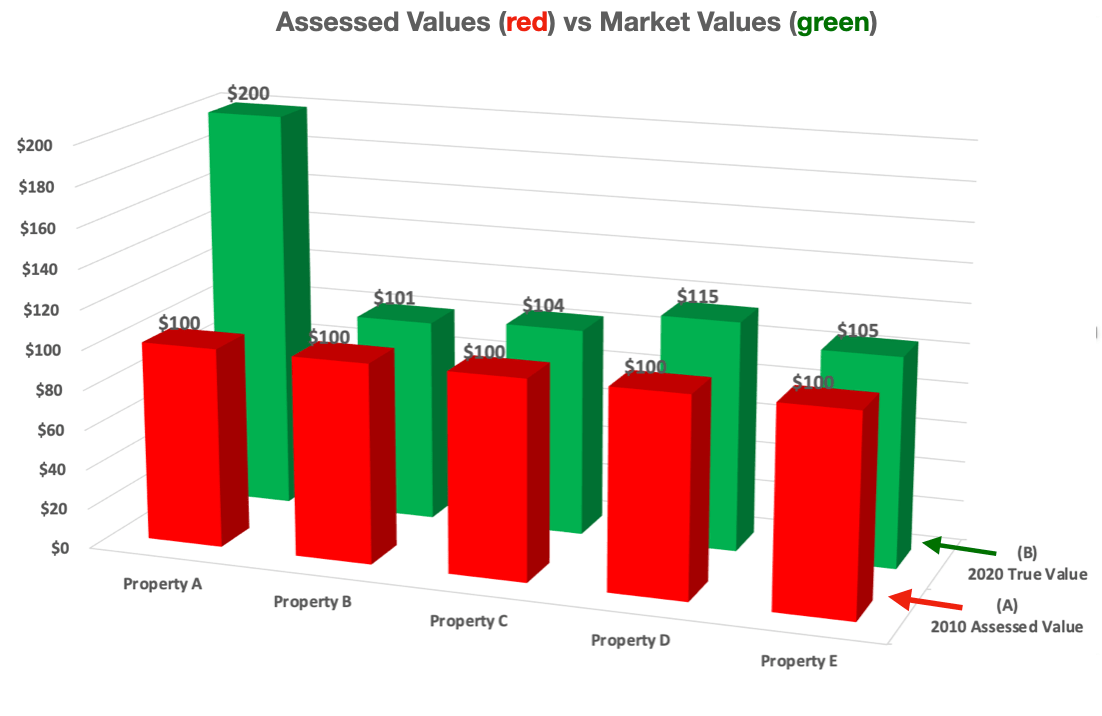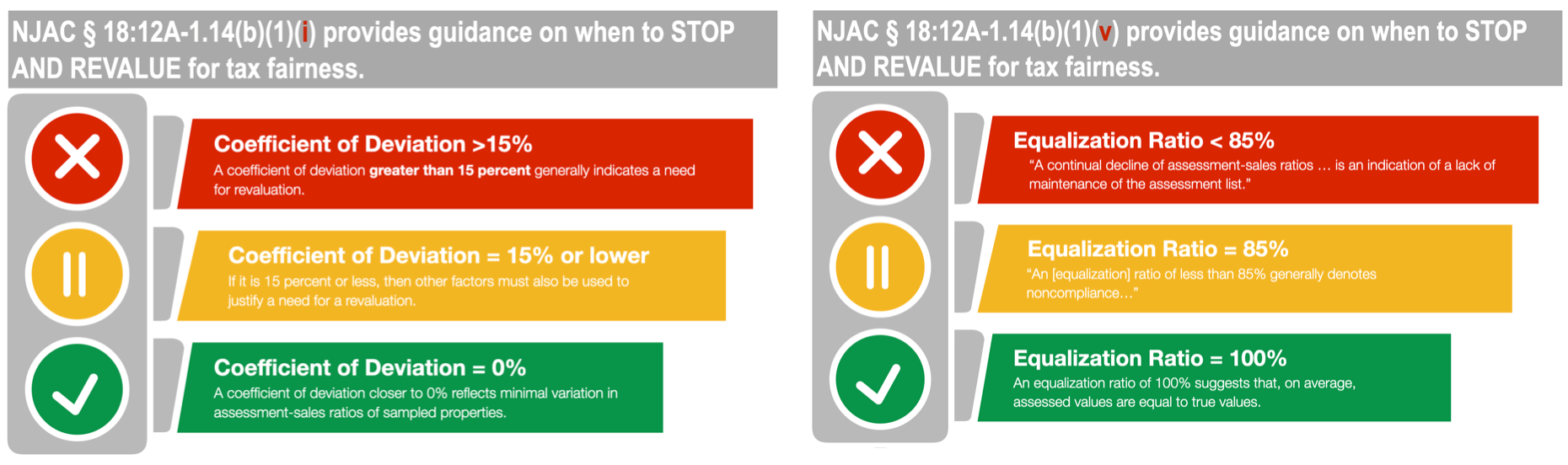# NJ Property Revaluation Dashboard, Part 1

This part of a CivicParent NJ Revaluation series.  Please see PropertyRevaluation.org for additional posts and analysis.

Tax fairness, at its core, depends on assessed values equaling market values. When these values grow disconnected – which can happen when real estate market values fluctuate away from static assessed values on record in the tax office – unfairness can grow. This “tax unfairness” is quantified each year on a per-municipality basis and NJ state law can help us understand and quantify the nuance. Specifically: N.J.A.C. § 18:12A-1.14(1) includes 12 criteria for revaluation, including two thresholds for potential tax unfairness:

• An equalization ratio below 85%
• A coefficient of deviation above 15%

To understand these two thresholds – how they are computed and what exactly they measure – we need to have a grounding in three key terms, each specific to your property :

• Assessed value is the value of your home in your tax record. This value is generally static – meaning it doesn’t change – over time. Assessed values get updated en masse throughout the entire city during a revaluation.
• True value is the current market value of the property. This value is changing all the time based on changes in the real estate market at large. True value is obtainable for free from a local licensed realtor.
• Assessment-sales ratio” measures the relationship of assessed to true value. It requires simple division to compute, i.e. Assessed Value / True Value = Assessment-Sales Ratio.

The equalization ratio is the average of all the assessment-sales ratios in the town.  And the coefficient of deviation measures the variance between individual assessment-sales ratios and the equalization ratio.

Let’s illustrate how these terms are applied with a simple example.

### A simple example to illustrate tax fairness math.

Imagine we have a sample town containing five houses, Properties “A” through “E”.

These five homes comprise the total tax base for the sample town. All five homes were assessed in 2010 for \$100, but by 2020 the true market values for each home had appreciated at varying rates.

• Property A appreciated from \$100 to \$200 (100% growth).
• Property B appreciated from \$100 to \$101 (1% growth).
• Property C appreciated from \$100 to \$104 (4% growth).
• Property D appreciated from \$100 to \$115 (15% growth).
• Property E appreciated from \$100 to \$105 (5% growth).

Here’s how the assessed values and market values look, graphed:The assessment-sales ratio is the first step to understanding the disconnectedness between assessed and market values, and comparing the disconnect among homes. To compute the ratio, we divide the home’s assessed value by its market value. The assessment-sales ratio data for our six sample homes are:

• Property A: 50% (\$100 / \$200)
• Property B: 99% (\$100 / \$101)
• Property C: 96% (\$100 / \$104)
• Property D: 87% (\$100 / \$115)
• Property E: 95% (\$100 / \$105)
• The average (aka the equalization ratio): 85% computed as: (50% + 99% + 96% + 87% + 95%) / 5)

Here’s how the assessment-sales ratios look, graphed:Now that we know our individual data, let’s compute the citywide data:

1-The equalization ratio is the average of the individual ratios.

2-The coefficient of deviation, on the other hand, measures the variance, or spread, of the individual ratios from the equalization ratio. Another way to think about this measure is: how close or far away are the individual ratios from the average…and…are there any major swings outside the ‘average’ range? The coefficient of deviation is computed by dividing the standard deviation into the equalization ratio:

Standard Deviation (50%, 99%, 96%, 87%, 95%) / Equalization Ratio of 85.47%

Looking at our sample town, we can see that there is some variance among all properties, but Property “A” has the most variance, sitting well outside the “pack” that is huddled above the average of 85%.  Property “A” is driving the coefficient of deviation UP

Our sample town has a coefficient of deviation of 21%.  [If you’re interested in the math I have a google sheet here with the data, chart, and computations noted above.  This is obviously an extremely simple scenario – deliberately so – to illustrate the basic concept. If you want to learn more about the real-world process, you can refer to the NJ administrative code § 18:12A-1.14 and the NJ Assessor’s Handbook.]

As I noted at the top of this post, NJ computes the equalization ratio and coefficient of deviation for every municipality and the thresholds they provide are like traffic lights:

• An equalization ratio less than 85% suggests enough disconnect between assessed and true values, on average, to explore revaluation.
• A coefficient of deviation of greater than 15% suggests enough variation – or spread – to explore revaluation.

If we were to apply NJ’s property tax law to our sample town, we’d have a case for exploring revaluation because (a) the coefficient of deviation of 21% is greater than 15% (beyond the threshold codified in N.J.A.C. § 18:12A-1.14(i)) and (b) the equalization ratio is hovering at the threshold of 85% per N.J.A.C. § 18:12A-1.14(i).### Find your town’s equalization ratio and coefficient of deviation.

The NJ Division of Taxation publishes equalization ratios and coefficients of deviation each year (see the data here and here). I combined the two datasets into one table and plotted each town with the equalization ratio charted on the Y-axis and the coefficient of deviation charted on the X axis. By graphing these two measures together, we can visualize a “Revaluation Dashboard” that indicates if a town is in:

theGreen” Zone:

The upper-left quadrant is the “green” zone, containing towns and cities that have an equalization ratio greater than 85% and a coefficient of deviation less than 15%.  These metrics suggest assessed values that are still both uniform and relatively close to true, or market, value.

the “Orange” Zone:

The lower left quadrant contains towns and cities with an equalization ratio of less than 85% meaning they are below the threshold codified in NJAC § 18:12A-1.14(b)(1)(v). This lower average suggests an increasing disconnect – on average – between assessed and true values.

The upper right quadrant contains towns and cities with a coefficient of deviation of greater than 15% meaning they are above the threshold codified in NJAC § 18:12A-1.14(b)(1)(i).  This higher variation suggests increasing non-uniformity between assessed and true values. Meaning, more properties like “A” above which are spread further out from the mean.

the “Red Zone”:

The lower right quadrant contains towns and cities triggering the thresholds established in both NJAC § 18:12A-1.14(b)(1)(v) and NJAC § 18:12A-1.14(b)(1)(i). These measures suggest both an increasing disconnect between assessed and true values AND an increasing variation, or spread.

This data is also in the data table below; you can filter by county, municipality, equalization ratio, or coefficient of deviation. Links to the source data are below the table.

### Brigid D'Souza

Scroll to top